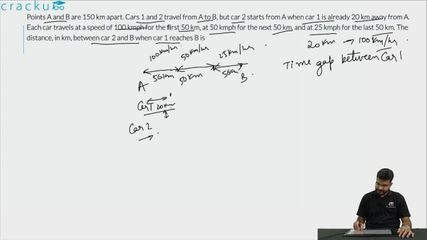Question 83

# Points A and B are 150 km apart. Cars 1 and 2 travel from A to B, but car 2 starts from A when car 1 is already 20 km away from A. Each car travels at a speed of 100 kmph for the ﬁrst 50 km, at 50 kmph for the next 50 km, and at 25 kmph for the last 50 km. The distance, in km, between car 2 and B when car 1 reaches B is

Solution

Time taken to cover first 50 km at 100 km/hr = $$\frac{1}{2}$$ hr.
Time taken to cover second 50 km at 50 km/hr = 1 hr.
Time taken to cover last 50 km at 25 km/hr = 2 hr.
When car 2 starts, car 1 has already covered 20 km.
So, time taken by car 1 to reach B after car 1 starts = total time - time required to travel first 20 km
= 3 hr 30 min - 12 min = 3 hr 18 min
Distance travelled by car 2 = (50 + 50 + 45) = 145 km
Distance from B = (150 - 145) km = 5 km

Hence, 5 is the correct answer.

### View Video Solution